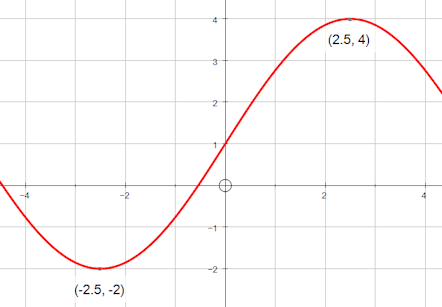Exam-Style Questions on Circular functions

Problems on Circular functions adapted from questions set in previous Mathematics exams.

1.

IB Standard

Consider the graph of $$f(x)=a\sin(b(x+c))+12$$, for $$0\le x\le 24$$.

The graph has a maximum at (8, 22) and a minimum at (18, 2).

(a) Find the value of $$a$$.

(b) Find the value of $$b$$.

(c) Find the value of $$c$$.

(d) Solve $$f(x)=5$$.

2.

IB Standard

The diagram shows part of the graph of $$y=asinbx+c$$ with a minimum at $$(-2.5,-2)$$ and a maximum at $$(2.5,4)$$.(a) Find $$a$$.

(b) Find $$b$$.

(c) Find $$c$$.

3.

IB Standard

The Big Wheel at Fantasy Fun Fayre rotates clockwise at a constant speed completing 15 rotations every hour. The wheel has a diameter of 90 metres and the bottom of the wheel is 6 metres above the ground.A cabin starts at the bottom of the wheel with the top of the cabin 6m above the ground.

(a) Find the greatest height of the top of the cabin reaches as the wheel rotates.

After $$t$$ minutes, the height $$h(t)$$ metres above the ground of the top of a cabin is given by the function $$h(t)=51-a\cos bt$$.

(b) Find the period of $$h(t)$$

(c) Find the value of $$b$$.

(d) Find the value of $$a$$.

(e) Sketch the graph of $$h(t)$$ , for $$0\le t\le 5$$.

(f) In one rotation of the wheel, find the probability that a randomly selected seat is at least 70 metres above the ground. Give your answer to two decimal places.

4.

IB Standard

The population of sheep on a ranch is modelled by the function $$P(t)= 65 \sin(0.4t-3.2)+450$$, where t is in months, and $$t=1$$ corresponds to 1st January 2015.

(a) Find the number of sheep on the ranch on 1st July 2015.

(b) Find the rate of change of the sheep population on 1st July 2015.

(c) Explain your answer to part (b) with reference to the sheep population size on 1st July2015.

5.

IB Standard

Let $$f(x)=\sin ( \frac {\pi}{4}x) + \cos ( \frac {\pi}{4}x)$$, for $$-4\le x \le 4$$

(a) Sketch the graph of $$f$$.

(b) Find the values of $$x$$ where the function is decreasing.

(c) The function $$f$$ can also be written in the form $$f(x)=a\sin ( \frac {\pi}{4}(x+c))$$ where $$a\in \mathbf R$$ and $$0 \le c \le 2$$. Find the value of $$a$$ and $$c$$.

6.

A-Level

The height above the ground, H metres, of a passenger on a Ferris wheel t minutes after the wheel starts turning, is modelled by the following equation:

$$H = k – 8\cos (60t)° + 5\sin (60t)°$$

where k is a constant.

(a) Express $$H$$ in the form $$H = k - R \cos(60t + a)°$$ where $$R$$ and $$a$$ are constants to be found ($$0° \lt a \lt 90°$$).

(b) Given that the initial height of the passenger above the ground is 2 metres, find a complete equation for the model.

(c) Hence find the maximum height of the passenger above the ground.

(d) Find the time taken for the passenger to reach the maximum height on the fifth cycle. (Solutions based entirely on graphical or numerical methods are not acceptable.)

(e) It is decided that, to increase profits, the speed of the wheel is to be increased. How would you adapt the equation of the model to reflect this increase in speed?

If you would like space on the right of the question to write out the solution try this Thinning Feature. It will collapse the text into the left half of your screen but large diagrams will remain unchanged.

The exam-style questions appearing on this site are based on those set in previous examinations (or sample assessment papers for future examinations) by the major examination boards. The wording, diagrams and figures used in these questions have been changed from the originals so that students can have fresh, relevant problem solving practice even if they have previously worked through the related exam paper.

The solutions to the questions on this website are only available to those who have a Transum Subscription.

Exam-Style Questions Main Page

Search for exam-style questions containing a particular word or phrase:

To search the entire Transum website use the search box in the grey area below.